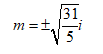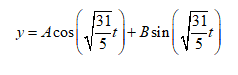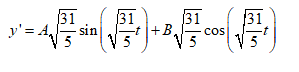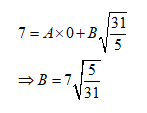Question
1 views

Find y as a function of t if

5y′′+31y=0

y(0)=4,y′(0)=7
y(t)=

check_circle

Solving the given differential equation

The given differential equation is

5y''+31y=0   ......(1)

and the initial condition is y(0)=4 and y'(0)=7

To get the auxiliary equation we replace y by 1 and y'' by m2

So, the auxiliary equation is

5m2+31=0

The roots of the above equation areso, the solution of the differential equation is......(1)

where A and B are arbitrary constants

Find the arbitrary constants

Using y(0)=4 we get

4=A*1+B*0

A=4

Differentiating (1) with respect to t we getNow using y'(0)=7 we get...

### Want to see the full answer?

See Solution

#### Want to see this answer and more?

Solutions are written by subject experts who are available 24/7. Questions are typically answered within 1 hour.*

See Solution
*Response times may vary by subject and question.
Tagged in